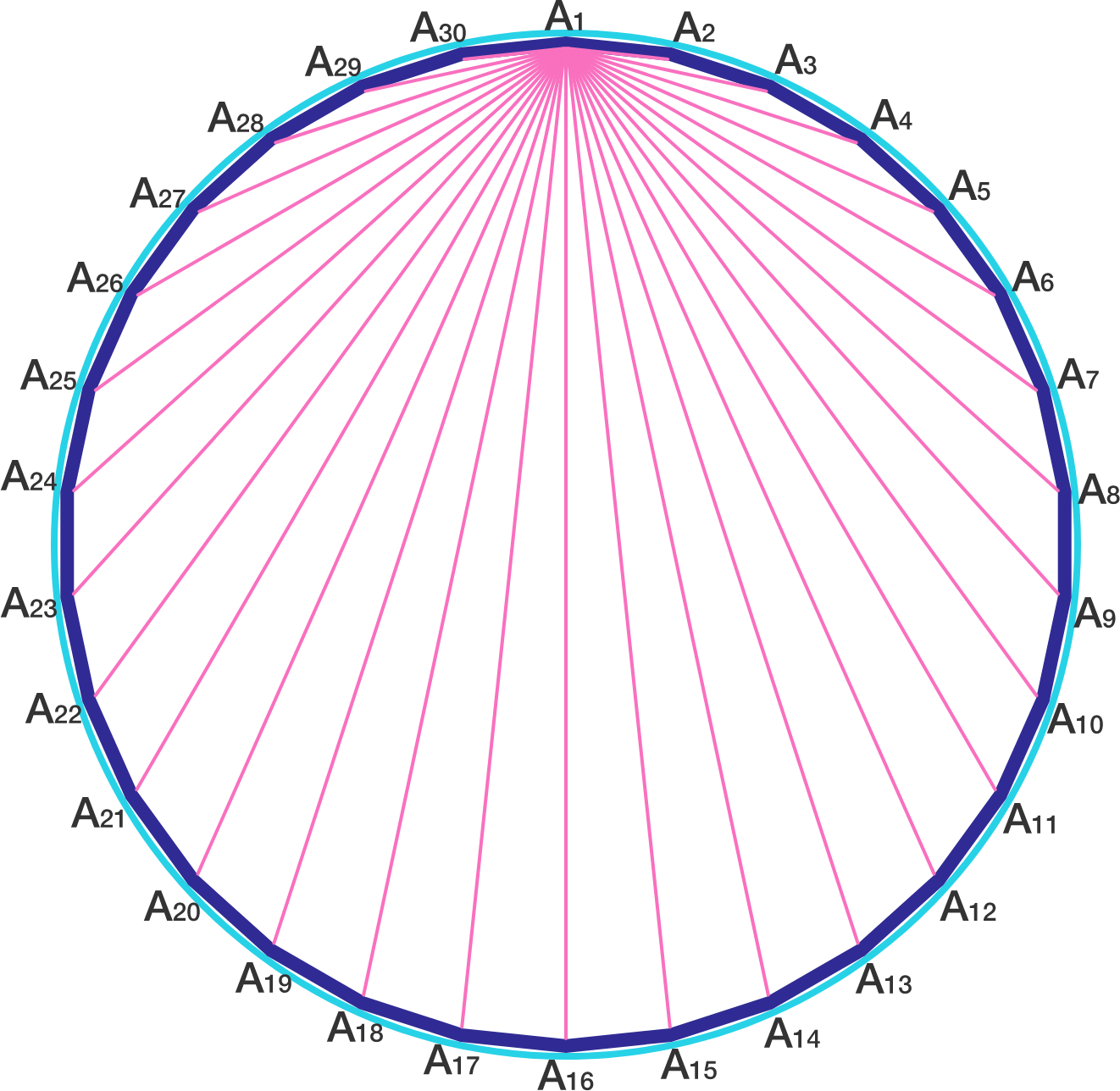# A regular 30-gon

Geometry Level 5

If $A_1 , A_2 , A_3 , \ldots , A_{30}$ are the vertices of a regular 30-gon inscribed in a unit circle, then find

${|A_1 A_2|}^2 + {|A_1 A_3|}^2 + {|A_1 A_4|}^2 + \cdots + {|A_1 A_{30}|}^2 .$×